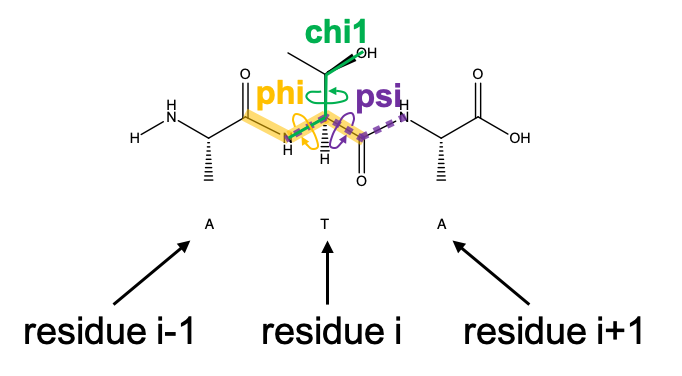This notebook contains material from PyRosetta; content is available on Github.

# Getting spatial features from a Pose¶

Keywords: conformation(), bond_length(), AtomID, atom_index()

In [ ]:
# Notebook setup
import sys
!pip install pyrosettacolabsetup
import pyrosettacolabsetup
pyrosettacolabsetup.mount_pyrosetta_install()
print ("Notebook is set for PyRosetta use in Colab.  Have fun!")

In [ ]:
from pyrosetta import *
init()


From previous section: Make sure you are in the directory with the pdb files:

cd google_drive/My\ Drive/student-notebooks/

In [ ]:
pose = pose_from_pdb("inputs/5tj3.pdb")

In :
from IPython.display import Image
Image('./Media/dihedral-final.png',width='500')

Out:Pose objects make it easy to access angles, distances, and torsions for analysis. Lets take a look at how to get backbone torsions first.

In :
#resid = "get the pose residue number for chain A:res 28 using the pdb2pose function"
### BEGIN SOLUTION
resid = pose.pdb_info().pdb2pose('A', 28)
### END SOLUTION

In :
print("phi:", pose.phi(resid))
print("psi:", pose.psi(resid))
print("chi1:", pose.chi(1, resid))

phi: -149.17513487055064
psi: 151.30037995499168
chi1: -82.850785668982


Say we want to find the length of the $N$-$C_\alpha$ and $C_\alpha$-$C$ bonds for residue A:28 from the PDB file. We can use a couple approaches. The first involves using the bond length in the Conformation class, which stores some info on protein geometry. Take a look at some of the methods in the Conformation class using tab completion.

In :
conformation = pose.conformation()
# do some tab completion here to explore the Conformation class methods
#conformation.


Look at the documentation for the method conformation.bond_length below. Remember using the ?

In :
### BEGIN SOLUTION
# ?conformation.bond_length
### END SOLUTION

Object conformation.bond_length not found.


To use the bond_length method in the Conformation class, it looks like we'll need to make AtomID objects. We can do this using an atom index and residue ID as follows:

In :
# Double Check: does resid contain the Pose numbering or PDB numbering?
res_28 = pose.residue(resid)
N28 = AtomID(res_28.atom_index("N"), resid)
CA28 = AtomID(res_28.atom_index("CA"), resid)
C28 = AtomID(res_28.atom_index("C"), resid)

# try printing out an AtomID object!

In :
### BEGIN SOLUTION
print(N28)
### END SOLUTION

 atomno= 1 rsd= 5


As usual, if you did not know how to construct an AtomID, you could check the documentation using ?AtomID.

Now we can compute the bond lengths:

In :
print(pose.conformation().bond_length(N28, CA28))
print(pose.conformation().bond_length(CA28, C28))

1.456100614655453
1.5184027792387658


Alternatively, we can compute bond lengths ourselves starting from the xyz coordinates of the atoms.

The method xyz of Residue returns a Vector class. The Vector class has various useful builtin methods including computing dot products, cross products, and norms. Through operator overloading in the Vector class, you can just subtract and add vector objects and they will manipulate the corresponding vectors appropriately.

In :
N_xyz = res_28.xyz("N")
CA_xyz = res_28.xyz("CA")
C_xyz = res_28.xyz("C")
N_CA_vector = CA_xyz - N_xyz
CA_C_vector = CA_xyz - C_xyz
print(N_CA_vector.norm())
print(CA_C_vector.norm())

1.456100614655453
1.5184027792387658


Thankfully, the two approaches for computing distances check out!

Note: Not all bond lengths, angles, and torsions will be accessible using the Conformation object. That is because the Conformation object stores only the subset it needs to generate xyz locations for the atoms in the pose. The most stable way to get this information is to compute it using the xyz Cartesian coordinate vectors as a starting point.

In [ ]:



This notebook includes some concepts and exercises from: